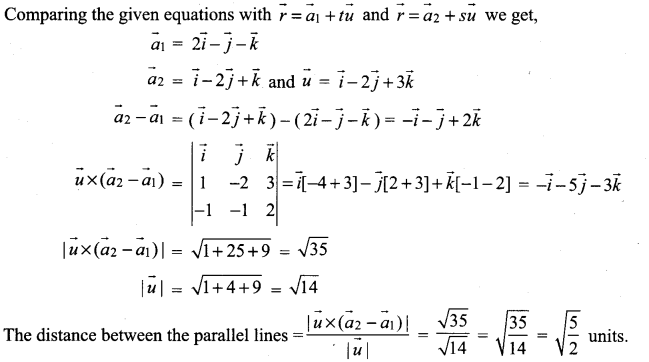## Tamilnadu Samacheer Kalvi 12th Maths Solutions Chapter 6 Applications of Vector Algebra Ex 6.5

Question 1.
Find the parametric form of vector equation and Cartesian equations of a straight line passing through (5, 2, 8) and is perpendicular to the straight lines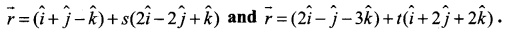Solution:∴ This’ vector is perpendicular to both the given straight lines.
∴ The required straight line is
$$\vec{r}=\vec{a}+t(\vec{b} \times \vec{d})$$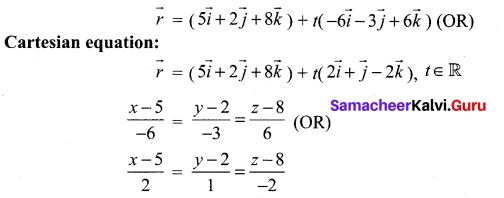Question 2.
Show that the lines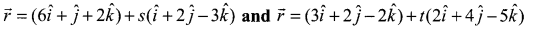are skew lines and hence find the shortest distance between them.
Solution: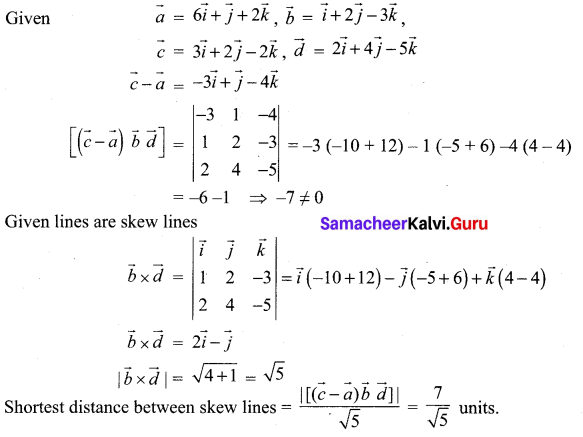Question 3.
If the two lines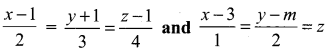intersect at a point, find the value of m.
Solution: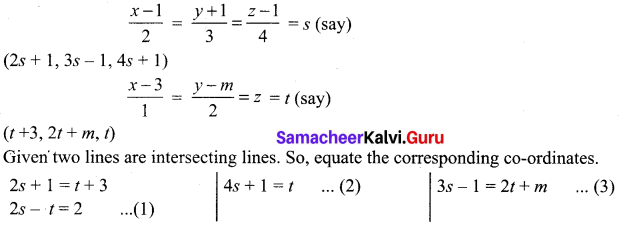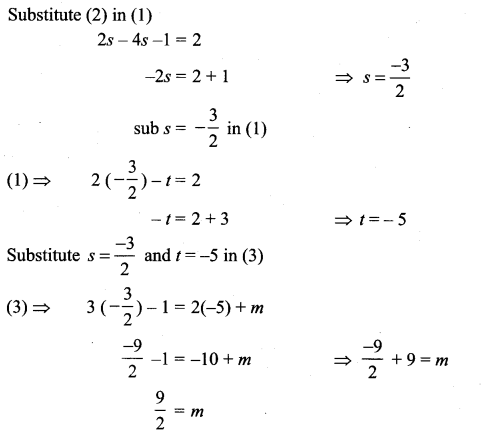Question 4.
Show that the lines $$\frac{x-3}{3}=\frac{y-3}{-1}$$, z – 1 = 0 and $$\frac{x-6}{2}=\frac{z-1}{3}$$, y – 2 = 0 intersect. Also find the point of intersection
Solution: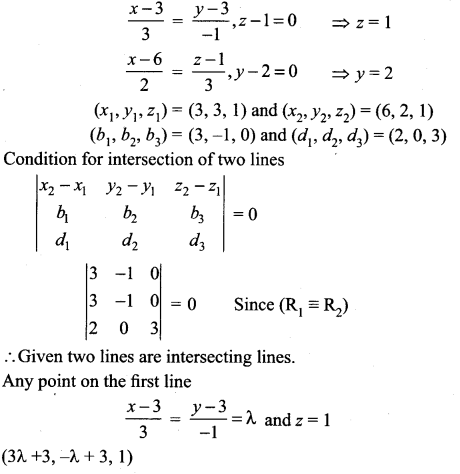Any point on the Second line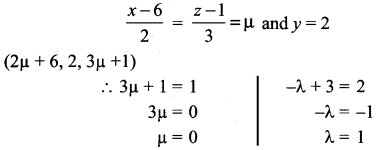∴ The required point of intersection is (6, 2, 1)

Question 5.
Show that the straight lines x + 1 = 2y = -12z and x = y + 2 = 6z – 6 are skew and hence find the shortest distance between them.
Solution: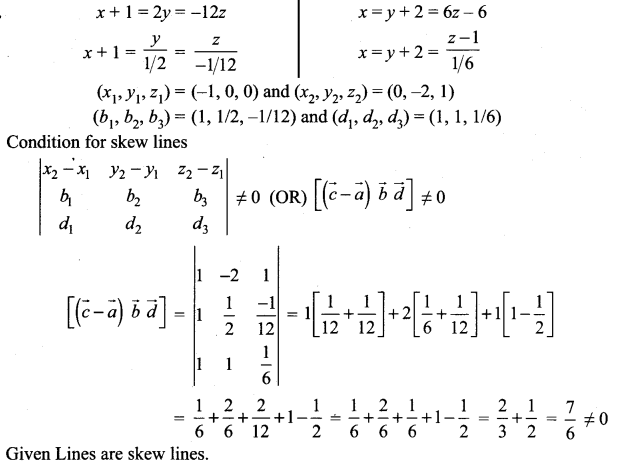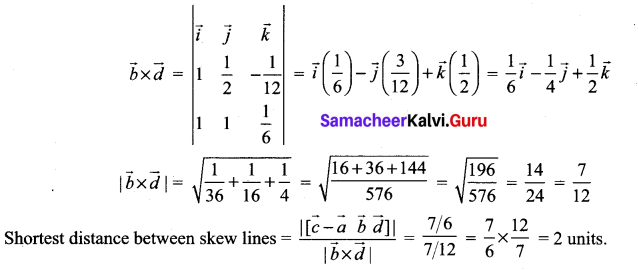Question 6.
Find the parametric form of vector equation of the straight line passing through (-1, 2, 1) and parallel to the straight line $$\vec{r}=(2 \hat{i}+3 \hat{j}-\hat{k})+t(\hat{i}-2 \hat{j}+\hat{k})$$ and hence find the shortest distance between the lines.
Solution: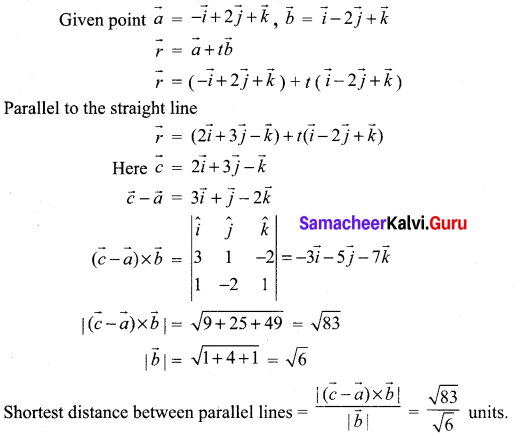Question 7.
Find the foot of the perpendicular drawn from the point (5, 4, 2) to the line $$\frac{x+1}{2}=\frac{y-3}{3}=\frac{z-1}{-1}$$. Also, find the equation of the perpendicular.
Solution: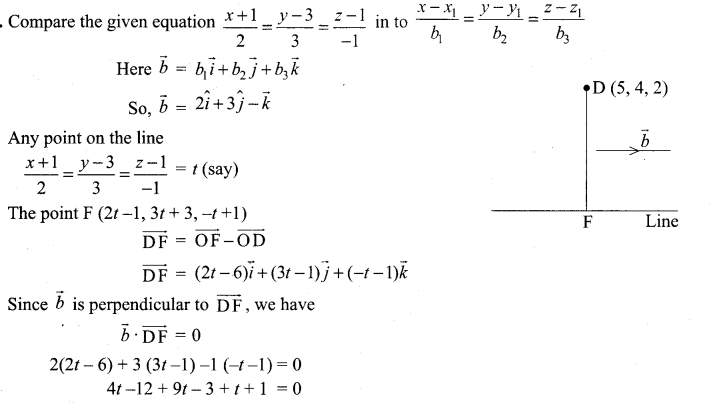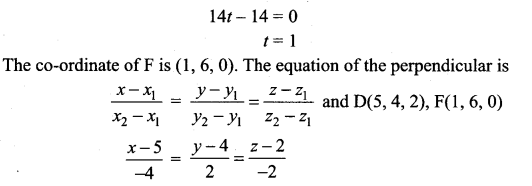### Samacheer Kalvi 12th Maths Solutions Chapter 6 Applications of Vector Algebra Ex 6.5 Additional Problems

Question 1.
Find the shortest distance between the parallel line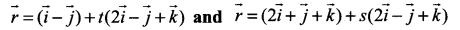Solution: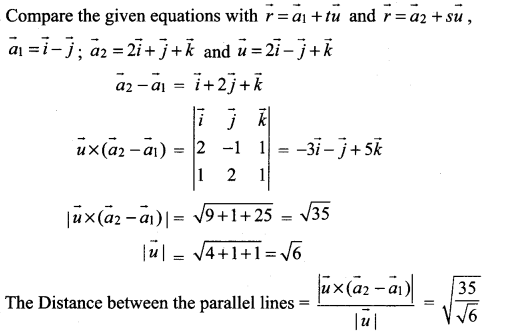Question 2.
Show that the two lines $$\vec{r}=(\vec{i}-\vec{j})+t(2 \vec{i}+\vec{k})$$ and $$\vec{r}=(2 \vec{i}-\vec{j})+s(\vec{i}+\vec{j}-\vec{k})$$ skew lines and find the distance between them.
Solution: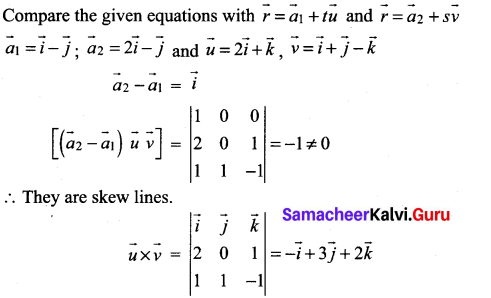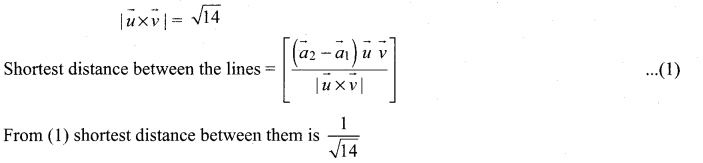Question 3.
Show that the lines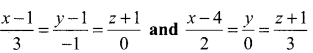intersect and hence find the point of intersection
Solution: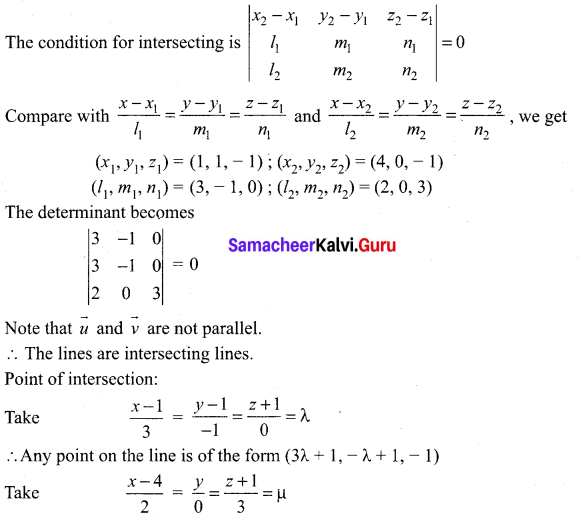Any point on this line is of the form (2µ + 4, 0, 3µ – 1)
Since they are intersecting, for some λ, µ
(3λ + 1, – λ + 1,- 1) = (2µ + 4, 0, 3µ – 1) ⇒ λ = 1 and µ = 0
To find the point of intersection either take λ = 1 or µ = 0
∴ The point of intersection is (4, 0, – 1),Question 4.
Find the shortest distance between the skew lines.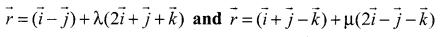Solution:
Compare the given equation with $$\vec{r}=\vec{a}_{1}+t \vec{u}$$ and $$\vec{r}=\vec{a}_{2}+s \vec{v}$$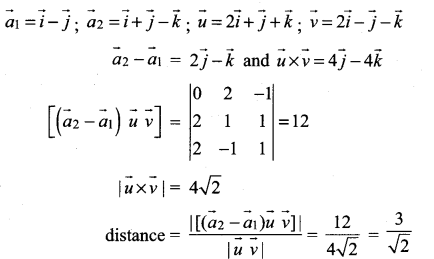Question 5.
Find the shortest distance between the parallel lines.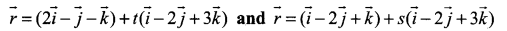Solution: